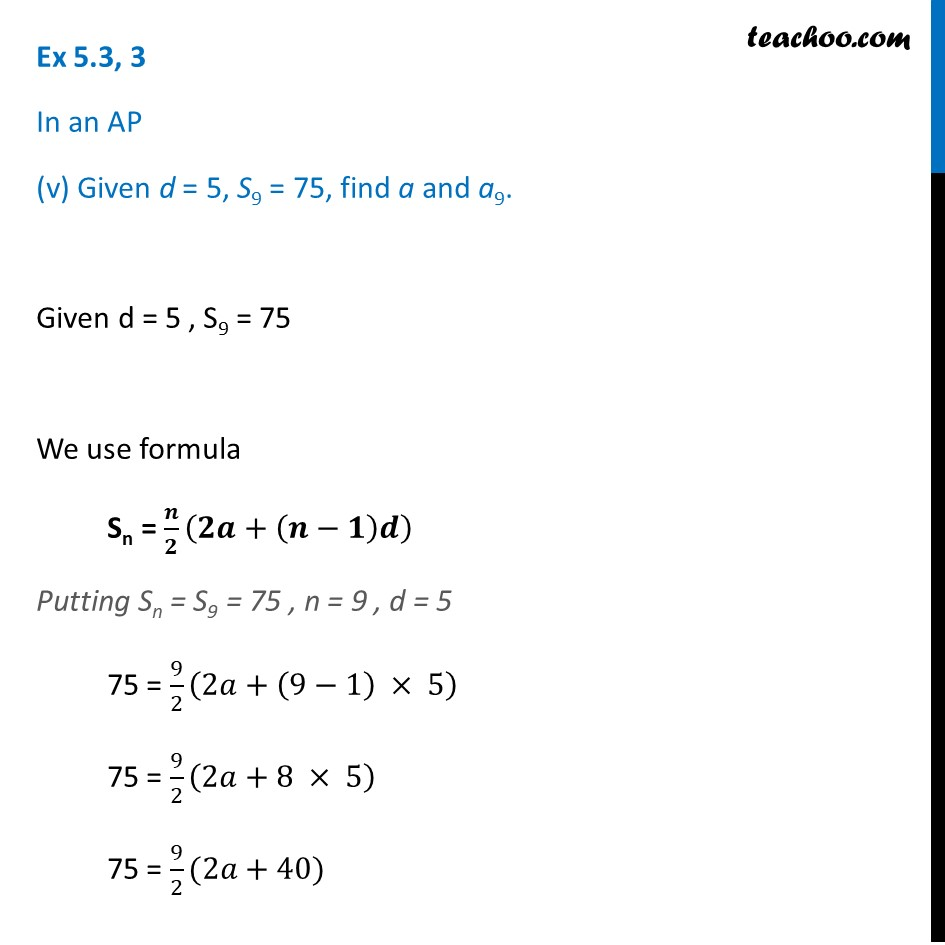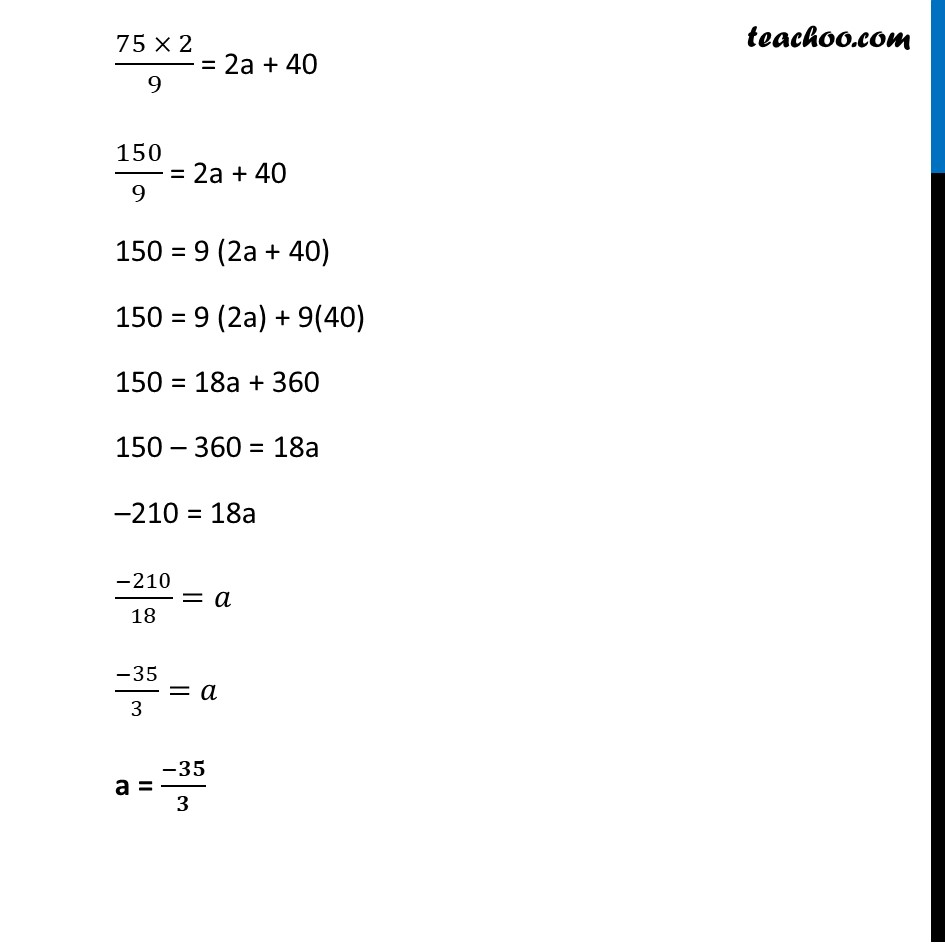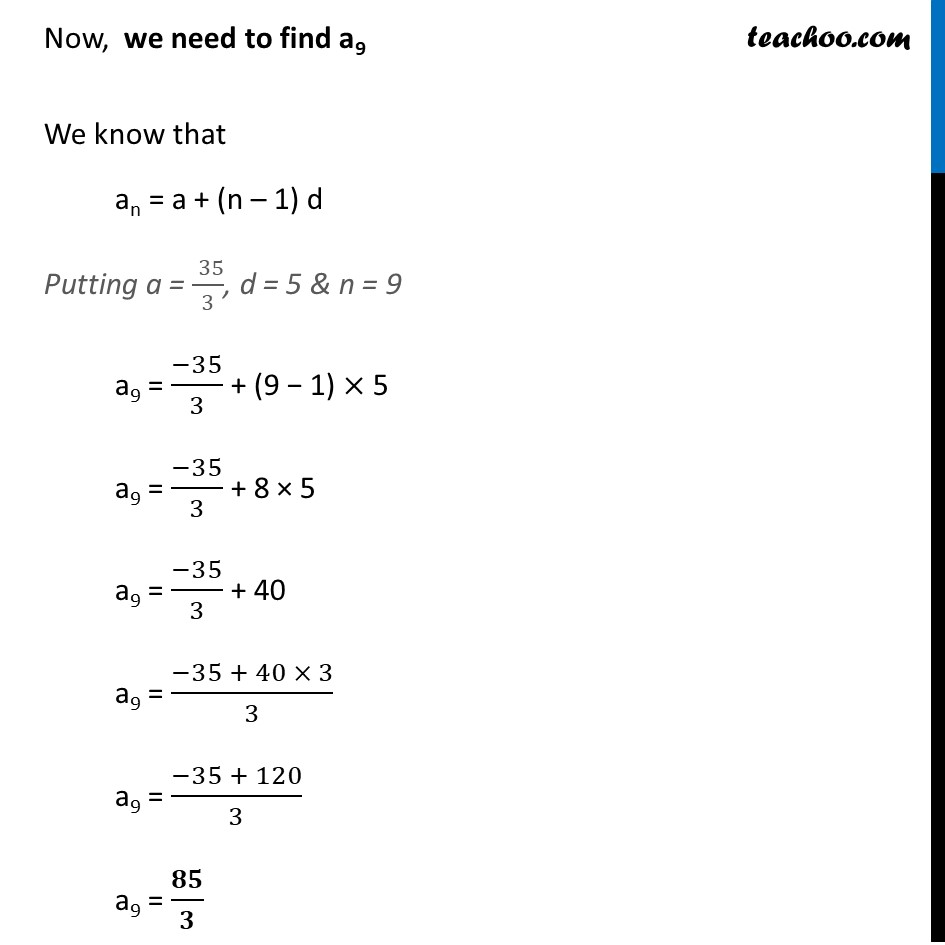1. Chapter 5 Class 10 Arithmetic Progressions (Term 2)
2. Serial order wise
3. Ex 5.3

Transcript

Ex 5.3, 3 In an AP (v) Given d = 5, S9 = 75, find a and a9. Given d = 5 , S9 = 75 We use formula Sn = 𝒏/𝟐 (𝟐𝒂+(𝒏−𝟏)𝒅) Putting Sn = S9 = 75 , n = 9 , d = 5 75 = 9/2 (2𝑎+(9−1) × 5) 75 = 9/2 (2𝑎+8 × 5) 75 = 9/2(2𝑎+40) (75 × 2)/9 = 2a + 40 150/9 = 2a + 40 150 = 9 (2a + 40) 150 = 9 (2a) + 9(40) 150 = 18a + 360 150 – 360 = 18a –210 = 18a (−210)/18=𝑎 (−35)/3=𝑎 a = (−𝟑𝟓)/𝟑 Now, we need to find a9 We know that an = a + (n – 1) d Putting a = ( 35)/3, d = 5 & n = 9 a9 = (−35)/3 + (9 − 1) × 5 a9 = (−35)/3 + 8 × 5 a9 = (−35)/3 + 40 a9 = (−35 + 40 × 3)/3 a9 = (−35 + 120)/3 a9 = 𝟖𝟓/𝟑